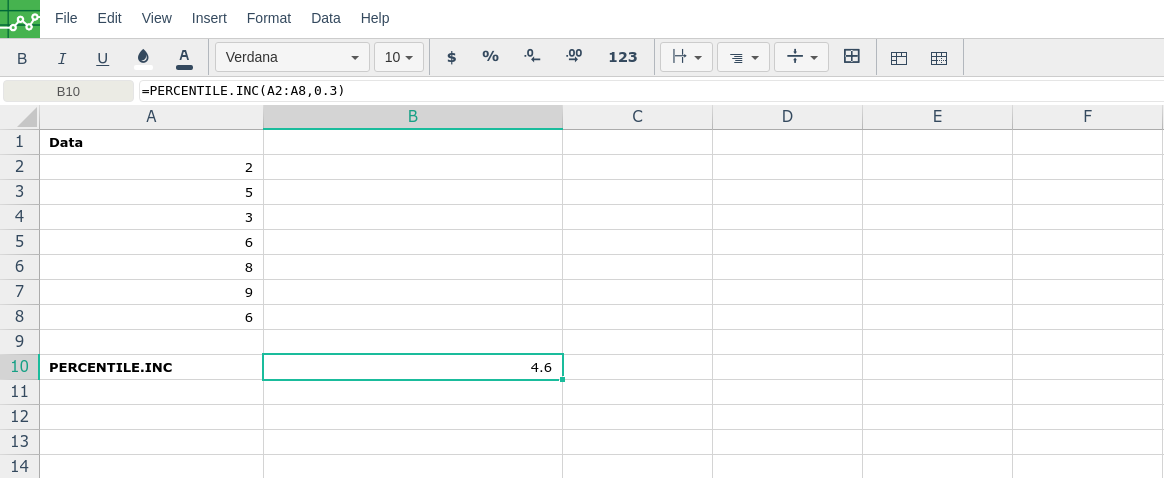# PERCENTILE.INC

### Definition

Returns the k-th percentile of values in a range, where k is in the range 0..1, inclusive.

You can use this function to establish a threshold of acceptance. For example, you can decide to examine candidates who score above the 90th percentile.

### Syntax

PERCENTILE.INC(array,k)

The PERCENTILE.INC function syntax has the following arguments:

• Array     Required. The array or range of data that defines relative standing.

• K     Required. The percentile value in the range 0..1, inclusive.

### Remarks

• If array is empty, PERCENTILE.INC returns the #NUM! error value.

• If k is nonnumeric, PERCENTILE.INC returns the #VALUE! error value.

• If k is < 0 or if k > 1, PERCENTILE.INC returns the #NUM! error value.

• If k is not a multiple of 1/(n - 1), PERCENTILE.INC interpolates to determine the value at the k-th percentile.

###Error.

User does not have sufficient privileges to access this Content